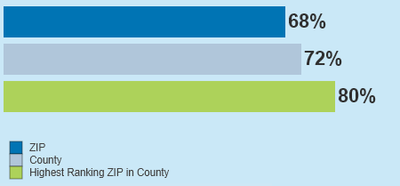# QlikView Creating Analytics

Discussion Board for collaboration related to Creating Analytics for QlikView.

Announcements
Leverage your QlikView investment to modernize BI – see how! Join Group
cancel
Showing results for
Did you mean:
HighlightedCreator

## Highest Rate of a ZIP in County - How to add to Chart

Hi All,

I have a chart in which I would like to display a rate for a selected ZIP and county, and the highest rate ZIP in the county selected. See below mock-up:My data is structured in a way that I can use the following expressions for Zip and County (assuming Zip and County are both required selections):

Zip rate bar:

Count(Distinct {<Numerator={'1'}>} Key)/Count(Distinct {<Denominator={'1'}>} Key)

County rate bar:

Count(Distinct {<Zip,Numerator={'1'}>} Key)/Count(Distinct {<Zip,Denominator={'1'}>} Key)

What logic would I need to add the green bar above - the Zip in the selected county with the highest rate? Currently I am not using a dimension to get the other two bars to appear.

1 Solution

Accepted Solutions
HighlightedMVP

## Re: Highest Rate of a ZIP in County - How to add to Chart

May be this

``Max({<Zip>} Aggr(Count(DISTINCT {<Zip, Numerator={'1'}>} Key)/Count(DISTINCT {<Zip, Denominator={'1'}>} Key), County, Zip))``
9 Replies
HighlightedCreator

## Re: Highest Rate of a ZIP in County - How to add to Chart

@sunny_talwar any ideas on this one? You've been a great help with these types of questions! Thanks so much!

HighlightedPartner

## Re: Highest Rate of a ZIP in County - How to add to Chart

maybe this expression gives you the desired value

max(aggr(Count(Distinct {<Numerator={'1'}>} Key)/Count(Distinct {<Denominator={'1'}>} Key),Zip))

HighlightedCreator

## Re: Highest Rate of a ZIP in County - How to add to Chart

This works only when County is selected but Zip is not selected. However, both Zip and County will be selected. When Zip is selected, this expression shows the value of the selected Zip, not the highest Zip in the county (regardless of selections). Any thoughts on modifying this to work when a Zip is selected?

HighlightedCreator

## Re: Highest Rate of a ZIP in County - How to add to Chart

@hrlinderany ideas to resolve the above issue? Thanks so much for your help!

HighlightedMVP

## Re: Highest Rate of a ZIP in County - How to add to Chart

May be this

``Max({<Zip>} Aggr(Count(DISTINCT {<Zip, Numerator={'1'}>} Key)/Count(DISTINCT {<Zip, Denominator={'1'}>} Key), County, Zip))``
HighlightedCreator

## Re: Highest Rate of a ZIP in County - How to add to Chart

Thank you, @Sunny ! This was what I was looking for. A request just came in though to only consider ZIPs meeting a certain threshold (for example, a denominator >=100):

Count(Distinct {<Denominator={'1'}>} Key)>100

How would you add this to your expression to limit which ZIPs are being considered within the county? I tried to add the following IF() but it doesn't appear to work when a ZIP is selected. Thoughts on how to modify this? Thank you!!

Max({<Zip>} Aggr(IF(Count(Distinct {<Denominator={'1'}>} Key)>100,Count(DISTINCT {<Zip, Numerator={'1'}>} Key)/Count(DISTINCT {<Zip, Denominator={'1'}>} Key)), County, Zip))

HighlightedMVP

## Re: Highest Rate of a ZIP in County - How to add to Chart

May be this

``Max({<Zip>} Aggr(Count(DISTINCT {<Zip = {"=Count(DISTINCT {<Zip, Denominator={'1'}>} Key) > 100"}, Numerator={'1'}>} Key)/Count(DISTINCT {<Zip = {"=Count(DISTINCT {<Zip, Denominator={'1'}>} Key) > 100"}, Denominator={'1'}>} Key), County, Zip))``
HighlightedCreator

## Re: Highest Rate of a ZIP in County - How to add to Chart

This worked great, @sunny_talwar ! One more request this morning - if I wanted to add another requirement for which ZIPs are considered, how do I add this to the set analysis? In addition to the denominator >= 100, I have now also been requested to limit to only ZIPs with a data coverage of 10%+:

COUNT(DISTINCT {<Denominator={'1'}>} Key)/SUM(PopZipCnt) >=.10

How do I add this to the set analysis below to require this coverage threshold in addition to the denominator threshold already included?

Thank you so much!

HighlightedMVP

## Re: Highest Rate of a ZIP in County - How to add to Chart

May be this

``Max({<Zip>} Aggr(Count(DISTINCT {<Zip = {"=Count(DISTINCT {<Zip, Denominator={'1'}>} Key) > 100 and Count(DISTINCT {<Denominator={'1'}>} Key)/SUM(PopZipCnt) >=.10"}, Numerator={'1'}>} Key)/Count(DISTINCT {<Zip = {"=Count(DISTINCT {<Zip, Denominator={'1'}>} Key) > 100 and Count(DISTINCT {<Denominator={'1'}>} Key)/SUM(PopZipCnt) >=.10"}, Denominator={'1'}>} Key), County, Zip))``